# Artificial Neural Network

7 Dec 2017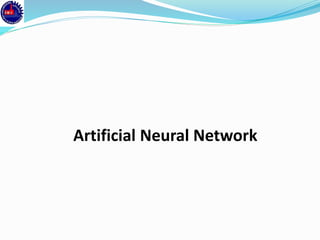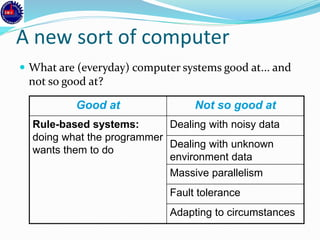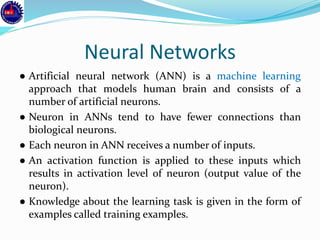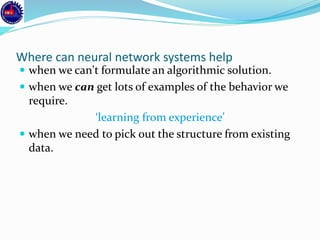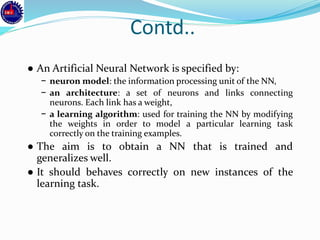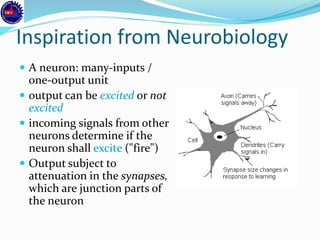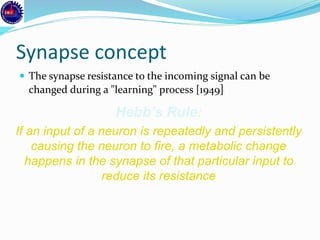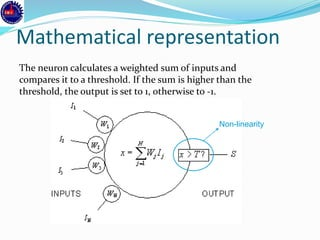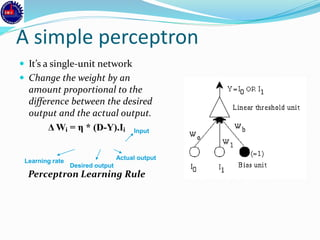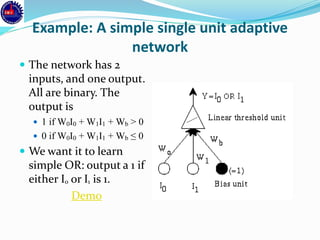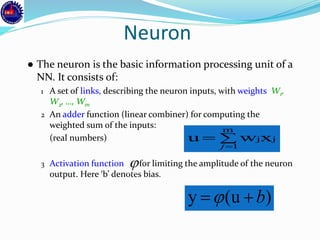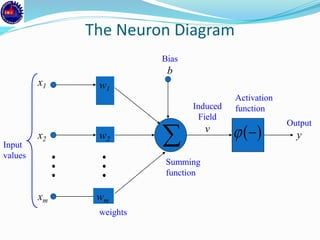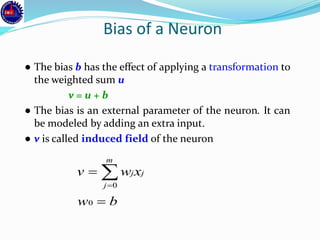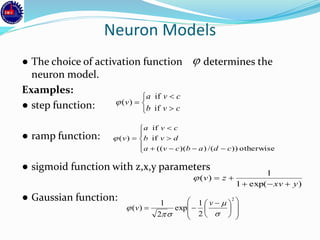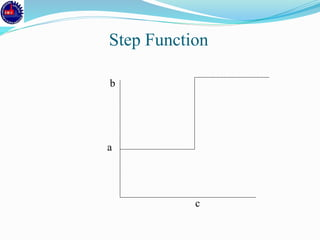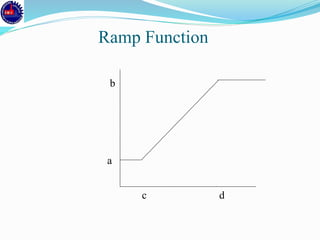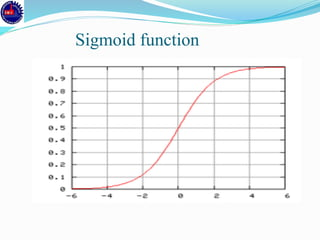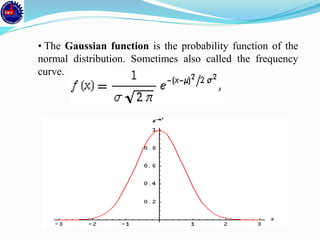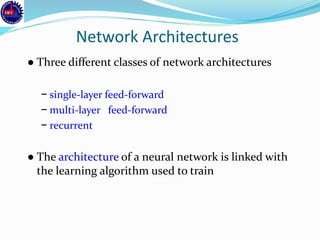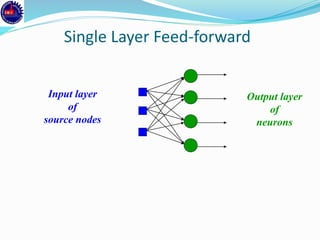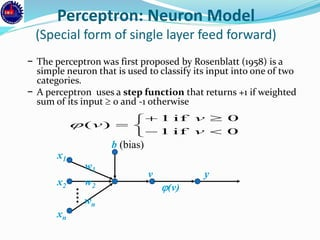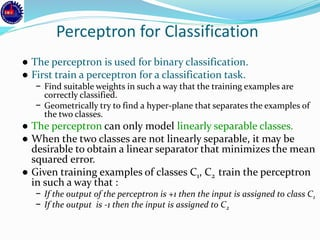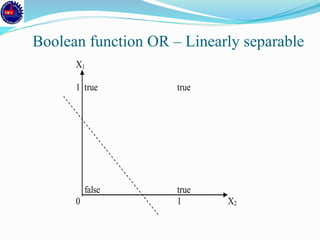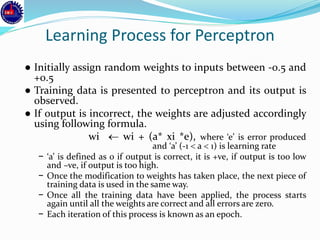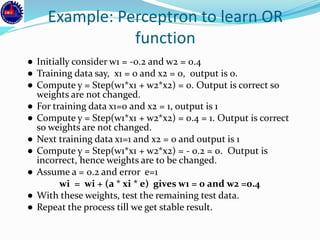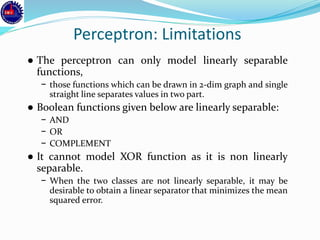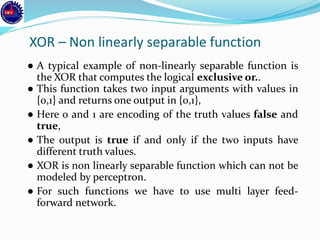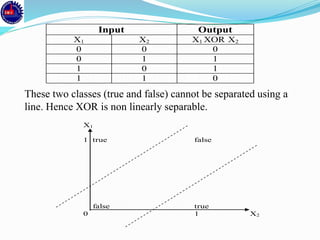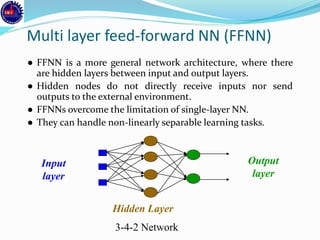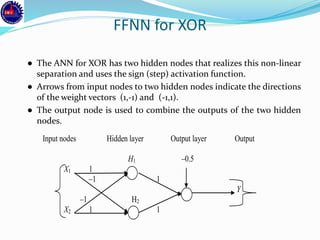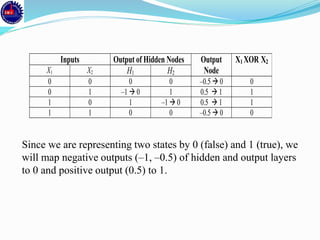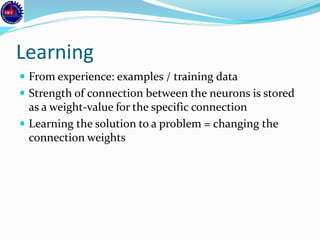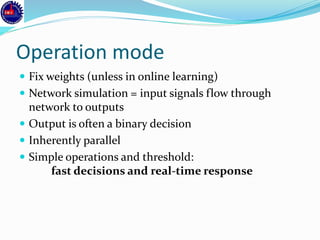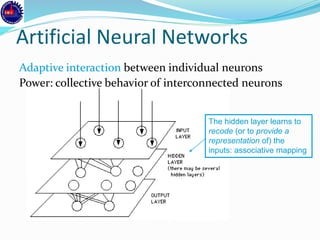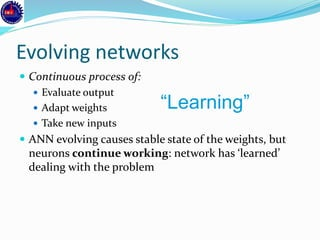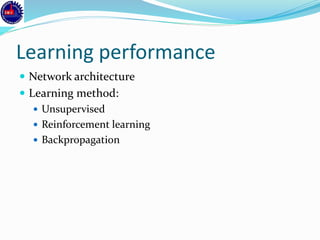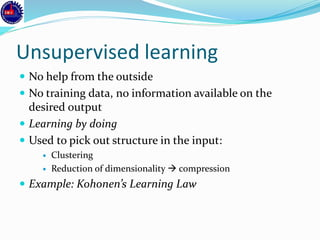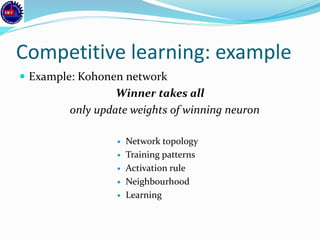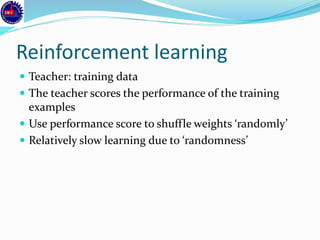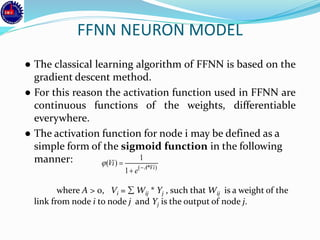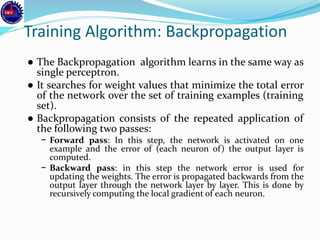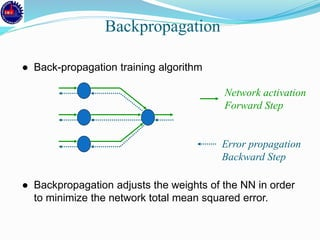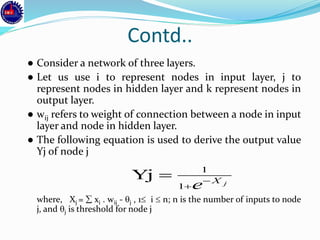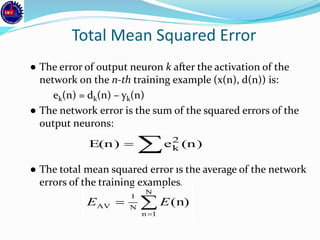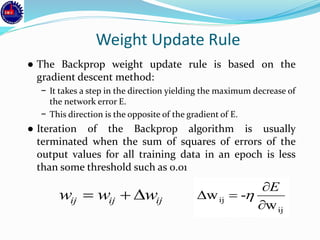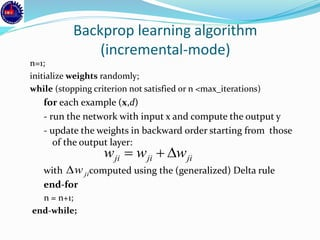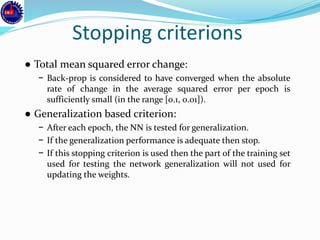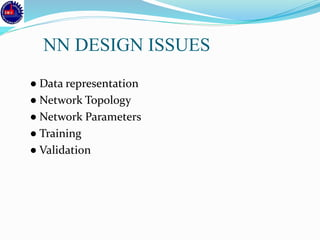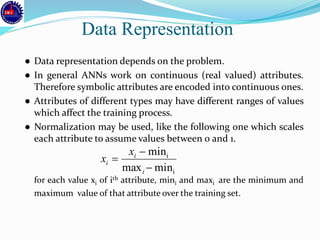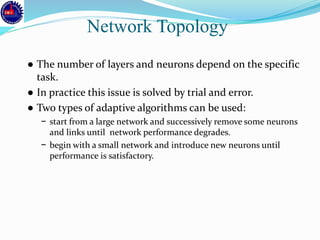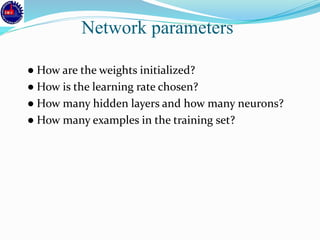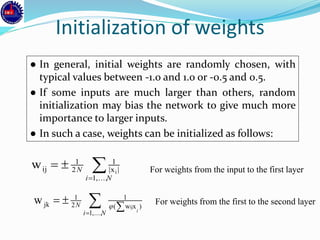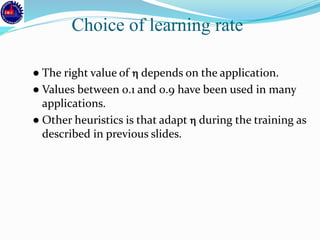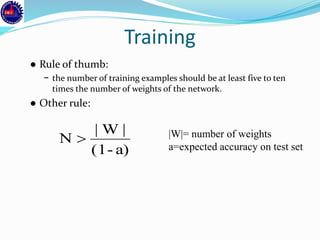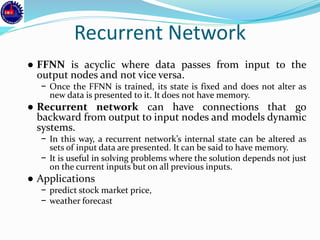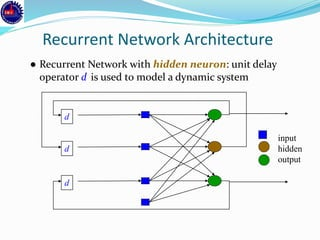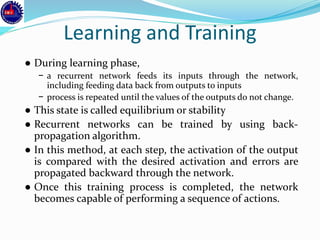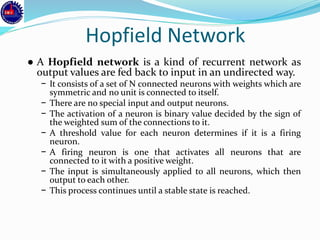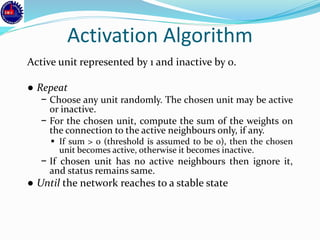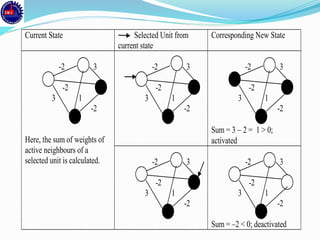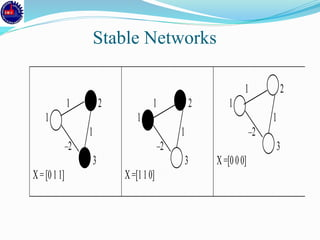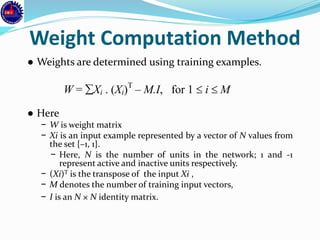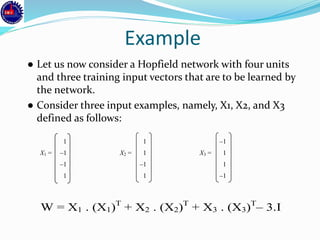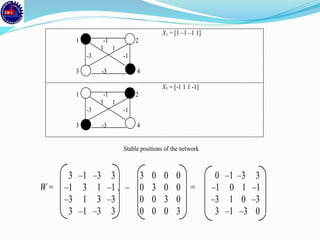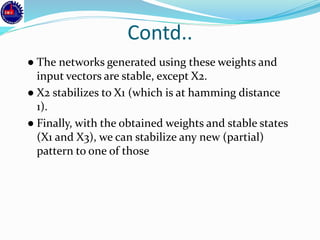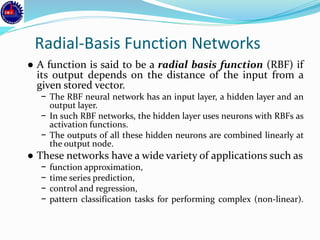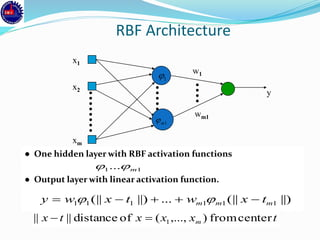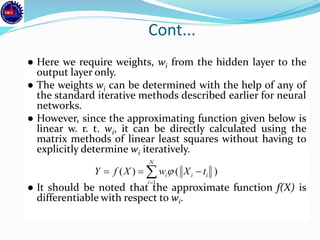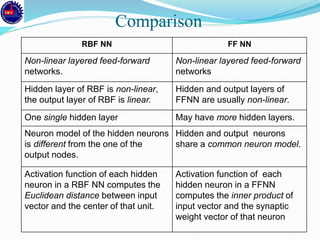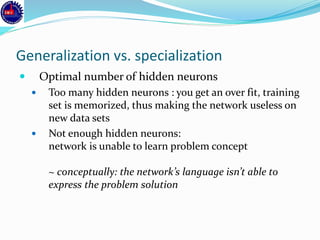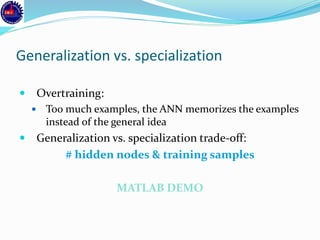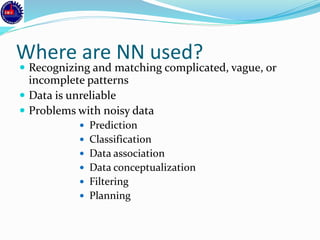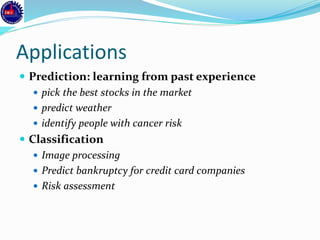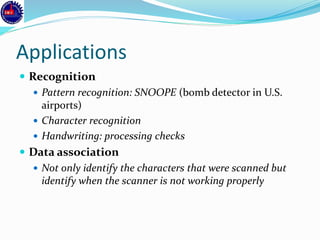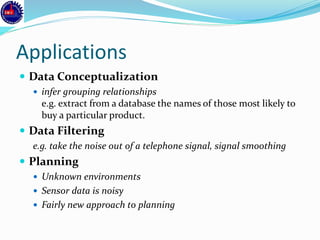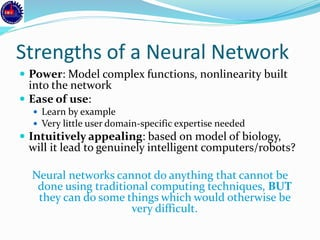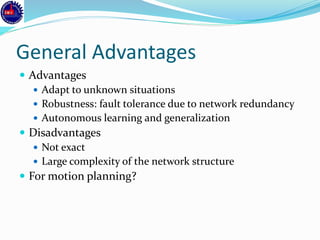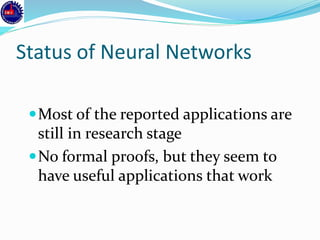1 sur 79

### Artificial Neural Network

• 2. A new sort of computer  What are (everyday) computer systems good at... and not so good at? Good at Not so good at Rule-based systems: doing what the programmer wants them to do Dealing with noisy data Dealing with unknown environment data Massive parallelism Fault tolerance Adapting to circumstances
• 3. Neural Networks ● Artificial neural network (ANN) is a machine learning approach that models human brain and consists of a number of artificial neurons. ● Neuron in ANNs tend to have fewer connections than biological neurons. ● Each neuron in ANN receives a number of inputs. ● An activation function is applied to these inputs which results in activation level of neuron (output value of the neuron). ● Knowledge about the learning task is given in the form of examples called training examples.
• 4. Where can neural network systems help  when we can't formulate an algorithmic solution.  when we can get lots of examples of the behavior we require. ‘learning from experience’  when we need to pick out the structure from existing data.
• 5. Contd.. ● An Artificial Neural Network is specified by: − neuron model: the information processing unit of the NN, − an architecture: a set of neurons and links connecting neurons. Each link has a weight, − a learning algorithm: used for training the NN by modifying the weights in order to model a particular learning task correctly on the training examples. ● The aim is to obtain a NN that is trained and generalizes well. ● It should behaves correctly on new instances of the learning task.
• 6. Inspiration from Neurobiology  A neuron: many-inputs / one-output unit  output can be excited or not excited  incoming signals from other neurons determine if the neuron shall excite ("fire")  Output subject to attenuation in the synapses, which are junction parts of the neuron
• 7. Synapse concept  The synapse resistance to the incoming signal can be changed during a "learning" process  Hebb’s Rule: If an input of a neuron is repeatedly and persistently causing the neuron to fire, a metabolic change happens in the synapse of that particular input to reduce its resistance
• 8. Mathematical representation The neuron calculates a weighted sum of inputs and compares it to a threshold. If the sum is higher than the threshold, the output is set to 1, otherwise to -1. Non-linearity
• 9. A simple perceptron  It’s a single-unit network  Change the weight by an amount proportional to the difference between the desired output and the actual output. Δ Wi = η * (D-Y).Ii Perceptron Learning Rule Learning rate Desired output Input Actual output
• 10. Example: A simple single unit adaptive network  The network has 2 inputs, and one output. All are binary. The output is  1 if W0I0 + W1I1 + Wb > 0  0 if W0I0 + W1I1 + Wb ≤ 0  We want it to learn simple OR: output a 1 if either I0 or I1 is 1. Demo
• 11. Neuron ● The neuron is the basic information processing unit of a NN. It consists of: 1 A set of links, describing the neuron inputs, with weights W1, W2, …, Wm 2 An adder function (linear combiner) for computing the weighted sum of the inputs: (real numbers) 3 Activation function for limiting the amplitude of the neuron output. Here ‘b’ denotes bias.   m 1 jjxwu j  )(uy b
• 13. Bias of a Neuron ● The bias b has the effect of applying a transformation to the weighted sum u v = u + b ● The bias is an external parameter of the neuron. It can be modeled by adding an extra input. ● v is called induced field of the neuron bw xwv j m j j    0 0
• 14. Neuron Models ● The choice of activation function determines the neuron model. Examples: ● step function: ● ramp function: ● sigmoid function with z,x,y parameters ● Gaussian function:                  2 2 1 exp 2 1 )(     v v )exp(1 1 )( yxv zv            otherwise))/())((( if if )( cdabcva dvb cva v       cvb cva v if if )(
• 18. • The Gaussian function is the probability function of the normal distribution. Sometimes also called the frequency curve.
• 19. Network Architectures ● Three different classes of network architectures − single-layer feed-forward − multi-layer feed-forward − recurrent ● The architecture of a neural network is linked with the learning algorithm used to train
• 20. Single Layer Feed-forward Input layer of source nodes Output layer of neurons
• 21. Perceptron: Neuron Model (Special form of single layer feed forward) − The perceptron was first proposed by Rosenblatt (1958) is a simple neuron that is used to classify its input into one of two categories. − A perceptron uses a step function that returns +1 if weighted sum of its input  0 and -1 otherwise x1 x2 xn w2 w1 wn b (bias) v y (v)       0if1 0if1 )( v v v
• 22. Perceptron for Classification ● The perceptron is used for binary classification. ● First train a perceptron for a classification task. − Find suitable weights in such a way that the training examples are correctly classified. − Geometrically try to find a hyper-plane that separates the examples of the two classes. ● The perceptron can only model linearly separable classes. ● When the two classes are not linearly separable, it may be desirable to obtain a linear separator that minimizes the mean squared error. ● Given training examples of classes C1, C2 train the perceptron in such a way that : − If the output of the perceptron is +1 then the input is assigned to class C1 − If the output is -1 then the input is assigned to C2
• 23. X1 1 true true false true 0 1 X2 Boolean function OR – Linearly separable
• 24. Learning Process for Perceptron ● Initially assign random weights to inputs between -0.5 and +0.5 ● Training data is presented to perceptron and its output is observed. ● If output is incorrect, the weights are adjusted accordingly using following formula. wi  wi + (a* xi *e), where ‘e’ is error produced and ‘a’ (-1  a  1) is learning rate − ‘a’ is defined as 0 if output is correct, it is +ve, if output is too low and –ve, if output is too high. − Once the modification to weights has taken place, the next piece of training data is used in the same way. − Once all the training data have been applied, the process starts again until all the weights are correct and all errors are zero. − Each iteration of this process is known as an epoch.
• 25. Example: Perceptron to learn OR function ● Initially consider w1 = -0.2 and w2 = 0.4 ● Training data say, x1 = 0 and x2 = 0, output is 0. ● Compute y = Step(w1*x1 + w2*x2) = 0. Output is correct so weights are not changed. ● For training data x1=0 and x2 = 1, output is 1 ● Compute y = Step(w1*x1 + w2*x2) = 0.4 = 1. Output is correct so weights are not changed. ● Next training data x1=1 and x2 = 0 and output is 1 ● Compute y = Step(w1*x1 + w2*x2) = - 0.2 = 0. Output is incorrect, hence weights are to be changed. ● Assume a = 0.2 and error e=1 wi = wi + (a * xi * e) gives w1 = 0 and w2 =0.4 ● With these weights, test the remaining test data. ● Repeat the process till we get stable result.
• 26. Perceptron: Limitations ● The perceptron can only model linearly separable functions, − those functions which can be drawn in 2-dim graph and single straight line separates values in two part. ● Boolean functions given below are linearly separable: − AND − OR − COMPLEMENT ● It cannot model XOR function as it is non linearly separable. − When the two classes are not linearly separable, it may be desirable to obtain a linear separator that minimizes the mean squared error.
• 27. XOR – Non linearly separable function ● A typical example of non-linearly separable function is the XOR that computes the logical exclusive or.. ● This function takes two input arguments with values in {0,1} and returns one output in {0,1}, ● Here 0 and 1 are encoding of the truth values false and true, ● The output is true if and only if the two inputs have different truth values. ● XOR is non linearly separable function which can not be modeled by perceptron. ● For such functions we have to use multi layer feed- forward network.
• 28. These two classes (true and false) cannot be separated using a line. Hence XOR is non linearly separable. Input Output X1 X2 X1 XOR X2 0 0 0 0 1 1 1 0 1 1 1 0 X1 1 true false false true 0 1 X2
• 29. Multi layer feed-forward NN (FFNN) ● FFNN is a more general network architecture, where there are hidden layers between input and output layers. ● Hidden nodes do not directly receive inputs nor send outputs to the external environment. ● FFNNs overcome the limitation of single-layer NN. ● They can handle non-linearly separable learning tasks. Input layer Output layer Hidden Layer 3-4-2 Network
• 30. FFNN for XOR ● The ANN for XOR has two hidden nodes that realizes this non-linear separation and uses the sign (step) activation function. ● Arrows from input nodes to two hidden nodes indicate the directions of the weight vectors (1,-1) and (-1,1). ● The output node is used to combine the outputs of the two hidden nodes. Input nodes Hidden layer Output layer Output H1 –0.5 X1 1 –1 1 Y –1 H2 X2 1 1
• 31. Inputs Output of Hidden Nodes Output Node X1 XOR X2 X1 X2 H1 H2 0 0 0 0 –0.5  0 0 0 1 –1  0 1 0.5  1 1 1 0 1 –1  0 0.5  1 1 1 1 0 0 –0.5  0 0 Since we are representing two states by 0 (false) and 1 (true), we will map negative outputs (–1, –0.5) of hidden and output layers to 0 and positive output (0.5) to 1.
• 32. Learning  From experience: examples / training data  Strength of connection between the neurons is stored as a weight-value for the specific connection  Learning the solution to a problem = changing the connection weights
• 33. Operation mode  Fix weights (unless in online learning)  Network simulation = input signals flow through network to outputs  Output is often a binary decision  Inherently parallel  Simple operations and threshold: fast decisions and real-time response
• 34. Artificial Neural Networks Adaptive interaction between individual neurons Power: collective behavior of interconnected neurons The hidden layer learns to recode (or to provide a representation of) the inputs: associative mapping
• 35. Evolving networks  Continuous process of:  Evaluate output  Adapt weights  Take new inputs  ANN evolving causes stable state of the weights, but neurons continue working: network has ‘learned’ dealing with the problem “Learning”
• 36. Learning performance  Network architecture  Learning method:  Unsupervised  Reinforcement learning  Backpropagation
• 37. Unsupervised learning  No help from the outside  No training data, no information available on the desired output  Learning by doing  Used to pick out structure in the input:  Clustering  Reduction of dimensionality  compression  Example: Kohonen’s Learning Law
• 38. Competitive learning: example  Example: Kohonen network Winner takes all only update weights of winning neuron  Network topology  Training patterns  Activation rule  Neighbourhood  Learning
• 39. Reinforcement learning  Teacher: training data  The teacher scores the performance of the training examples  Use performance score to shuffle weights ‘randomly’  Relatively slow learning due to ‘randomness’
• 40. FFNN NEURON MODEL ● The classical learning algorithm of FFNN is based on the gradient descent method. ● For this reason the activation function used in FFNN are continuous functions of the weights, differentiable everywhere. ● The activation function for node i may be defined as a simple form of the sigmoid function in the following manner: where A > 0, Vi =  Wij * Yj , such that Wij is a weight of the link from node i to node j and Yj is the output of node j. )*( 1 1 )( ViA e Vi   
• 41. Training Algorithm: Backpropagation ● The Backpropagation algorithm learns in the same way as single perceptron. ● It searches for weight values that minimize the total error of the network over the set of training examples (training set). ● Backpropagation consists of the repeated application of the following two passes: − Forward pass: In this step, the network is activated on one example and the error of (each neuron of) the output layer is computed. − Backward pass: in this step the network error is used for updating the weights. The error is propagated backwards from the output layer through the network layer by layer. This is done by recursively computing the local gradient of each neuron.
• 42. Backpropagation ● Back-propagation training algorithm ● Backpropagation adjusts the weights of the NN in order to minimize the network total mean squared error. Network activation Forward Step Error propagation Backward Step
• 43. Contd.. ● Consider a network of three layers. ● Let us use i to represent nodes in input layer, j to represent nodes in hidden layer and k represent nodes in output layer. ● wij refers to weight of connection between a node in input layer and node in hidden layer. ● The following equation is used to derive the output value Yj of node j where, Xj =  xi . wij - j , 1 i  n; n is the number of inputs to node j, and j is threshold for node j jX e   1 1 Yj
• 44. Total Mean Squared Error ● The error of output neuron k after the activation of the network on the n-th training example (x(n), d(n)) is: ek(n) = dk(n) – yk(n) ● The network error is the sum of the squared errors of the output neurons: ● The total mean squared error is the average of the network errors of the training examples. (n)eE(n) 2 k   N 1n N 1 AV (n)EE
• 45. Weight Update Rule ● The Backprop weight update rule is based on the gradient descent method: − It takes a step in the direction yielding the maximum decrease of the network error E. − This direction is the opposite of the gradient of E. ● Iteration of the Backprop algorithm is usually terminated when the sum of squares of errors of the output values for all training data in an epoch is less than some threshold such as 0.01 ijijij www  ij ij w -w    E 
• 46. Backprop learning algorithm (incremental-mode) n=1; initialize weights randomly; while (stopping criterion not satisfied or n <max_iterations) for each example (x,d) - run the network with input x and compute the output y - update the weights in backward order starting from those of the output layer: with computed using the (generalized) Delta rule end-for n = n+1; end-while; jijiji www  jiw
• 47. Stopping criterions ● Total mean squared error change: − Back-prop is considered to have converged when the absolute rate of change in the average squared error per epoch is sufficiently small (in the range [0.1, 0.01]). ● Generalization based criterion: − After each epoch, the NN is tested for generalization. − If the generalization performance is adequate then stop. − If this stopping criterion is used then the part of the training set used for testing the network generalization will not used for updating the weights.
• 48. ● Data representation ● Network Topology ● Network Parameters ● Training ● Validation NN DESIGN ISSUES
• 49. ● Data representation depends on the problem. ● In general ANNs work on continuous (real valued) attributes. Therefore symbolic attributes are encoded into continuous ones. ● Attributes of different types may have different ranges of values which affect the training process. ● Normalization may be used, like the following one which scales each attribute to assume values between 0 and 1. for each value xi of ith attribute, mini and maxi are the minimum and maximum value of that attribute over the training set. Data Representation i i minmax min    i i i x x
• 50. ● The number of layers and neurons depend on the specific task. ● In practice this issue is solved by trial and error. ● Two types of adaptive algorithms can be used: − start from a large network and successively remove some neurons and links until network performance degrades. − begin with a small network and introduce new neurons until performance is satisfactory. Network Topology
• 51. ● How are the weights initialized? ● How is the learning rate chosen? ● How many hidden layers and how many neurons? ● How many examples in the training set? Network parameters
• 52. Initialization of weights ● In general, initial weights are randomly chosen, with typical values between -1.0 and 1.0 or -0.5 and 0.5. ● If some inputs are much larger than others, random initialization may bias the network to give much more importance to larger inputs. ● In such a case, weights can be initialized as follows:   Ni N ,...,1 |x| 1 2 1 ij i w For weights from the input to the first layer For weights from the first to the second layer   Ni N i ,...,1 )xw( 1 2 1 jk ij w 
• 53. ● The right value of  depends on the application. ● Values between 0.1 and 0.9 have been used in many applications. ● Other heuristics is that adapt  during the training as described in previous slides. Choice of learning rate
• 54. Training ● Rule of thumb: − the number of training examples should be at least five to ten times the number of weights of the network. ● Other rule: |W|= number of weights a=expected accuracy on test seta)-(1 |W| N 
• 55. Recurrent Network ● FFNN is acyclic where data passes from input to the output nodes and not vice versa. − Once the FFNN is trained, its state is fixed and does not alter as new data is presented to it. It does not have memory. ● Recurrent network can have connections that go backward from output to input nodes and models dynamic systems. − In this way, a recurrent network’s internal state can be altered as sets of input data are presented. It can be said to have memory. − It is useful in solving problems where the solution depends not just on the current inputs but on all previous inputs. ● Applications − predict stock market price, − weather forecast
• 56. ● Recurrent Network with hidden neuron: unit delay operator d is used to model a dynamic system d d d Recurrent Network Architecture input hidden output
• 57. Learning and Training ● During learning phase, − a recurrent network feeds its inputs through the network, including feeding data back from outputs to inputs − process is repeated until the values of the outputs do not change. ● This state is called equilibrium or stability ● Recurrent networks can be trained by using back- propagation algorithm. ● In this method, at each step, the activation of the output is compared with the desired activation and errors are propagated backward through the network. ● Once this training process is completed, the network becomes capable of performing a sequence of actions.
• 58. Hopfield Network ● A Hopfield network is a kind of recurrent network as output values are fed back to input in an undirected way. − It consists of a set of N connected neurons with weights which are symmetric and no unit is connected to itself. − There are no special input and output neurons. − The activation of a neuron is binary value decided by the sign of the weighted sum of the connections to it. − A threshold value for each neuron determines if it is a firing neuron. − A firing neuron is one that activates all neurons that are connected to it with a positive weight. − The input is simultaneously applied to all neurons, which then output to each other. − This process continues until a stable state is reached.
• 59. Activation Algorithm Active unit represented by 1 and inactive by 0. ● Repeat − Choose any unit randomly. The chosen unit may be active or inactive. − For the chosen unit, compute the sum of the weights on the connection to the active neighbours only, if any. ▪ If sum > 0 (threshold is assumed to be 0), then the chosen unit becomes active, otherwise it becomes inactive. − If chosen unit has no active neighbours then ignore it, and status remains same. ● Until the network reaches to a stable state
• 60. Current State Selected Unit from current state Corresponding New State -2 3 -2 3 1 -2 -2 3 -2 3 1 -2 Sum = 3 – 2 = 1 > 0; activated -2 3 -2 3 1 -2 Here, the sum of weights of active neighbours of a selected unit is calculated. -2 3 -2 3 1 -2 -2 3 -2 3 1 -2 Sum = –2 < 0; deactivated
• 61. 1 2 1 1 –2 3 X= 1 2 1 1 –2 3 X= 1 2 1 1 –2 3 X= Stable Networks
• 62. Weight Computation Method ● Weights are determined using training examples. ● Here − W is weight matrix − Xi is an input example represented by a vector of N values from the set {–1, 1}. − Here, N is the number of units in the network; 1 and -1 represent active and inactive units respectively. − (Xi)T is the transpose of the input Xi , − M denotes the number of training input vectors, − I is an N × N identity matrix. W = Xi . (Xi)T – M.I, for 1  i  M
• 63. Example ● Let us now consider a Hopfield network with four units and three training input vectors that are to be learned by the network. ● Consider three input examples, namely, X1, X2, and X3 defined as follows: 1 1 –1 X1 = –1 X2 = 1 X3 = 1 –1 –1 1 1 1 –1 W = X1 . (X1)T + X2 . (X2)T + X3 . (X3)T – 3.I
• 64. 3 –1 –3 3 3 0 0 0 0 –1 –3 3 W = –1 3 1 –1 . – 0 3 0 0 = –1 0 1 –1 –3 1 3 –3 0 0 3 0 –3 1 0 –3 3 –1 –3 3 0 0 0 3 3 –1 –3 0 X1 = [1 –1 –1 1] 1 -1 2 3 1 -3 -1 3 -3 4 X3 = [-1 1 1 -1] 1 -1 2 3 1 -3 -1 3 -3 4 Stable positions of the network
• 65. Contd.. ● The networks generated using these weights and input vectors are stable, except X2. ● X2 stabilizes to X1 (which is at hamming distance 1). ● Finally, with the obtained weights and stable states (X1 and X3), we can stabilize any new (partial) pattern to one of those
• 66. Radial-Basis Function Networks ● A function is said to be a radial basis function (RBF) if its output depends on the distance of the input from a given stored vector. − The RBF neural network has an input layer, a hidden layer and an output layer. − In such RBF networks, the hidden layer uses neurons with RBFs as activation functions. − The outputs of all these hidden neurons are combined linearly at the output node. ● These networks have a wide variety of applications such as − function approximation, − time series prediction, − control and regression, − pattern classification tasks for performing complex (non-linear).
• 67. RBF Architecture ● One hidden layer with RBF activation functions ● Output layer with linear activation function. x2 xm x1 y wm1 w1 1 1m 11... m ||)(||...||)(|| 111111 mmm txwtxwy   txxxtx m centerfrom),...,(ofdistance|||| 1
• 68. Cont... ● Here we require weights, wi from the hidden layer to the output layer only. ● The weights wi can be determined with the help of any of the standard iterative methods described earlier for neural networks. ● However, since the approximating function given below is linear w. r. t. wi, it can be directly calculated using the matrix methods of linear least squares without having to explicitly determine wi iteratively. ● It should be noted that the approximate function f(X) is differentiable with respect to wi. )()( 1   N i iii tXwXfY 
• 69. RBF NN FF NN Non-linear layered feed-forward networks. Non-linear layered feed-forward networks Hidden layer of RBF is non-linear, the output layer of RBF is linear. Hidden and output layers of FFNN are usually non-linear. One single hidden layer May have more hidden layers. Neuron model of the hidden neurons is different from the one of the output nodes. Hidden and output neurons share a common neuron model. Activation function of each hidden neuron in a RBF NN computes the Euclidean distance between input vector and the center of that unit. Activation function of each hidden neuron in a FFNN computes the inner product of input vector and the synaptic weight vector of that neuron Comparison
• 70. Generalization vs. specialization  Optimal number of hidden neurons  Too many hidden neurons : you get an over fit, training set is memorized, thus making the network useless on new data sets  Not enough hidden neurons: network is unable to learn problem concept ~ conceptually: the network’s language isn’t able to express the problem solution
• 71. Generalization vs. specialization  Overtraining:  Too much examples, the ANN memorizes the examples instead of the general idea  Generalization vs. specialization trade-off: # hidden nodes & training samples MATLAB DEMO
• 72. Where are NN used?  Recognizing and matching complicated, vague, or incomplete patterns  Data is unreliable  Problems with noisy data  Prediction  Classification  Data association  Data conceptualization  Filtering  Planning
• 73. Applications  Prediction: learning from past experience  pick the best stocks in the market  predict weather  identify people with cancer risk  Classification  Image processing  Predict bankruptcy for credit card companies  Risk assessment
• 74. Applications  Recognition  Pattern recognition: SNOOPE (bomb detector in U.S. airports)  Character recognition  Handwriting: processing checks  Data association  Not only identify the characters that were scanned but identify when the scanner is not working properly
• 75. Applications  Data Conceptualization  infer grouping relationships e.g. extract from a database the names of those most likely to buy a particular product.  Data Filtering e.g. take the noise out of a telephone signal, signal smoothing  Planning  Unknown environments  Sensor data is noisy  Fairly new approach to planning
• 76. Strengths of a Neural Network  Power: Model complex functions, nonlinearity built into the network  Ease of use:  Learn by example  Very little user domain-specific expertise needed  Intuitively appealing: based on model of biology, will it lead to genuinely intelligent computers/robots? Neural networks cannot do anything that cannot be done using traditional computing techniques, BUT they can do some things which would otherwise be very difficult.
• 77. General Advantages  Advantages  Adapt to unknown situations  Robustness: fault tolerance due to network redundancy  Autonomous learning and generalization  Disadvantages  Not exact  Large complexity of the network structure  For motion planning?
• 78. Status of Neural Networks Most of the reported applications are still in research stage No formal proofs, but they seem to have useful applications that work
• 79. Thanks ! 233Dr. B. C. Roy Engg. College